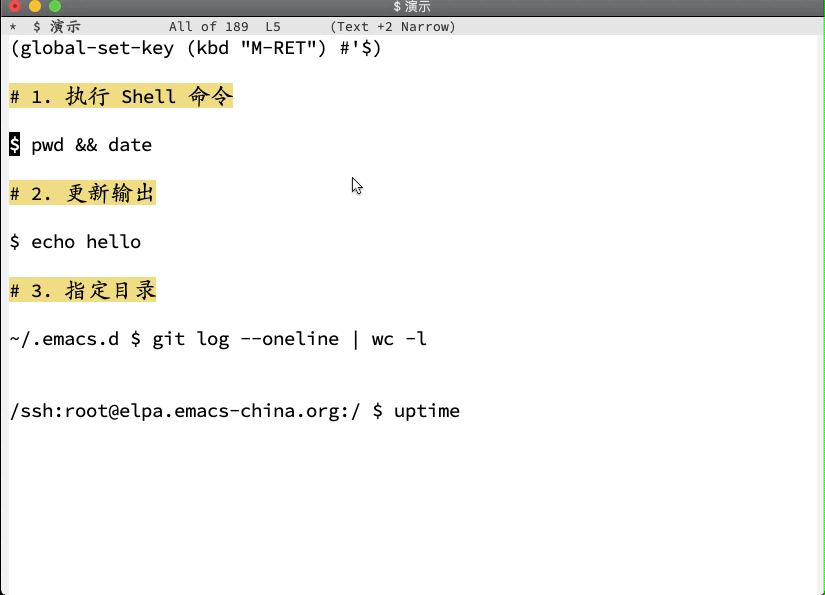# 受 Xiki 启发，想到一个执行 Shell 命令的方法``````(defun \$ (pattern)
"Run shell command in the current line and insert the output."
(interactive
(list (buffer-substring-no-properties
(line-beginning-position)
(line-end-position))))
(let (directory command)
(pcase pattern
((rx bos "\$ " (let cmd (1+ anything)) eos)
(setq directory default-directory
command cmd))
((rx bos (let dir (1+ (not (in " ")))) " \$ " (let cmd (1+ anything)) eos)
(setq directory dir
command cmd))
(_ (user-error "Unrecognized format, support '\$ date' and '~/.emacs.d \$ pwd'")))
(save-excursion
;; Delete old output
(delete-region (progn (forward-line)
(point))
(progn (while (get-text-property (point) '\$)
(forward-line))
(point)))
(unless (bolp) (insert "\n"))
(insert
(with-temp-buffer
(let ((default-directory directory))
(shell-command command t)

(propertize (buffer-string) '\$ t 'rear-nonsticky t)))))))
``````
6 个赞

1 个赞